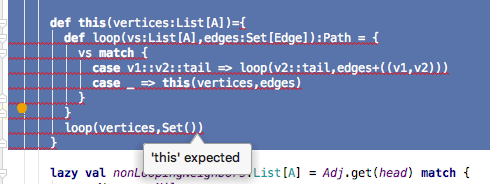# Local function inside class constructor `def this`

How does the `def this` work inside a class definition. It looks from the examples, that unlike other definitions, this one doesn’t depend on the object in tail position within the body of the definition. In my case, I call `this` in tail position, but both IntelliJ and the compiler that `this` is missing.

``````      def this(vertices:List[A])={
def loop(vs:List[A],edges:Set[Edge]):Path = {
vs match {
case v1::v2::tail => loop(v2::tail,edges+((v1,v2)))
case _ => this(vertices,edges)
}
}
loop(vertices,Set())
}
``````There are a lot of restrictions for secondary constructors. I try to never use them… I believe that a call to `this(...)` always has to be the first statement.
I estimate there’s a 99% chance you will want to transform this into a factory method in the companion object.

1 Like

AFAIR first call in a secondary constructor must be a call to some previous constructor (so ultimately that constructors call chain ends up in primary constructor).

As referred to in the other answers, the general idea of secondary constructor methods is to somehow build specific sets of arguments that you will use to call the primary constructor anyway.
So the secondary constructor will need to eventually call the main, passing all expected args.

The ratio is that you have better control of the integrity of class invariants by forcing all constructions to always pass through the same primary method.

@ivanopagano, yes that makes sense. However, it looks to me like the implementation of `this` in my original post does exactly that–it calls the main constructor in the tail position of a tail-recursive local function. However, the compiler sadly doesn’t like that arrangement very much.

Sorry, but tail recursion is probably not understood explicitly by the compiler.
I miss the necessary knowledge to appreciate the complexity of figuring it out in detail, but understanding recursion termination sounds much like solving the halting problem.
I guess that having the compiler being able to evaluate and guarantee generic recursion return values is not an easy feature.
The solution though, is pretty easy, and would be to bind the loop eventual value in a variable and pass that to an explicit call to the main constructor

``````     def this(vertices:List[A]) = {
@tailrec
def loop(vs:List[A],edges:Set[Edge]): Set[Edge] = {
vs match {
case v1::v2::tail => loop(v2::tail,edges+((v1,v2)))
case _ => edges
}
}
val edges = loop(vertices, Set())
this(vertices, edges)
}
``````

Edited to correct the refactoring

1 Like

Looking at it now (several days later) the logic is wrong. The purpose was to have a constructor which accepts just the vertices, but which re-calls the original constructer with the vertices and edges. In retrospect this is impossible, you can’t derive edges from vertices, but the other way around is possible (assuming now vertices are isolated).

Nevertheless, shouldn’t your proposed solution call `this` with two arguments, being the vertices and edges calculated by the `loop`? In your case it looks like the constructor `this(finalPath)` is being called with an actually already constructed object, i.e., the return value of `this(vertices,edges)`.

As @Jasper-M said, factory methods would probably be a best fit for the problem. Conventionally in Scala, factory methods are put in companion objects and are named `apply`. Scala often infers `apply` identifier, which means you can write `x(y)` instead of `x.apply(y)`. Example of factory methods here:

``````class MyClass(properState: Map[A, B]) {
// put fields, methods, but no secondary constructors here
}

object MyClass { // companion object
def apply(inputA: InputA): MyClass = {
// some code
new MyClass(constructedState)
}

def apply(inputB: InputB): MyClass = {
// some code
new MyClass(constructedState)
}
}

// thanks to `apply` inferring now we can write
MyClass(new InputA)